## mlcourse.ai – Open Machine Learning Course¶

Author: Egor Polusmak. Translated and edited by Yury Kashnitsky, and Yuanyuan Pao. This material is subject to the terms and conditions of the Creative Commons CC BY-NC-SA 4.0 license. Free use is permitted for any non-commercial purpose.

This is a static version of a Jupyter notebook. You can also check out the latest version in the course repository, the corresponding interactive web-based Kaggle Notebook or a video lecture.

# Topic 2. Visual data analysis in Python

## Part 1. Visualization: from Simple Distributions to Dimensionality Reduction

In the field of Machine Learning, data visualization is not just making fancy graphics for reports; it is used extensively in day-to-day work for all phases of a project.

To start with, visual exploration of data is the first thing one tends to do when dealing with a new task. We do preliminary checks and analysis using graphics and tables to summarize the data and leave out the less important details. It is much more convenient for us, humans, to grasp the main points this way than by reading many lines of raw data. It is amazing how much insight can be gained from seemingly simple charts created with available visualization tools.

Next, when we analyze the performance of a model or report results, we also often use charts and images. Sometimes, for interpreting a complex model, we need to project high-dimensional spaces onto more visually intelligible 2D or 3D figures.

All in all, visualization is a relatively fast way to learn something new about your data. Thus, it is vital to learn its most useful techniques and make them part of your everyday ML toolbox.

In this article, we are going to get hands-on experience with visual exploration of data using popular libraries such as pandas, matplotlib and seaborn.

## 1. Dataset¶

Before we get to the data, let's initialize our environment:

In :
# we don't like warnings
# you can comment the following 2 lines if you'd like to
import warnings

import numpy as np
import pandas as pd

warnings.filterwarnings("ignore")

# Matplotlib forms basis for visualization in Python
import matplotlib.pyplot as plt
# We will use the Seaborn library
import seaborn as sns

sns.set()

# Graphics in retina format are more sharp and legible
%config InlineBackend.figure_format = 'retina'


In the first article, we looked at the data on customer churn for a telecom operator. We will reload the same dataset into a DataFrame:

In :
df = pd.read_csv("../input/telecom_churn.csv")


To get to know our data, let's look at the first 5 entries using head():

In :
df.head()

Out:
State Account length Area code International plan Voice mail plan Number vmail messages Total day minutes Total day calls Total day charge Total eve minutes Total eve calls Total eve charge Total night minutes Total night calls Total night charge Total intl minutes Total intl calls Total intl charge Customer service calls Churn
0 KS 128 415 No Yes 25 265.1 110 45.07 197.4 99 16.78 244.7 91 11.01 10.0 3 2.70 1 False
1 OH 107 415 No Yes 26 161.6 123 27.47 195.5 103 16.62 254.4 103 11.45 13.7 3 3.70 1 False
2 NJ 137 415 No No 0 243.4 114 41.38 121.2 110 10.30 162.6 104 7.32 12.2 5 3.29 0 False
3 OH 84 408 Yes No 0 299.4 71 50.90 61.9 88 5.26 196.9 89 8.86 6.6 7 1.78 2 False
4 OK 75 415 Yes No 0 166.7 113 28.34 148.3 122 12.61 186.9 121 8.41 10.1 3 2.73 3 False

Here is the description of our features:

Name Description Value Type Statistical Type
State State abbreviation (like KS = Kansas) String Categorical
Account length How long the client has been with the company Numerical Quantitative
Area code Phone number prefix Numerical Categorical
International plan International plan (on/off) String, "Yes"/"No" Categorical/Binary
Voice mail plan Voicemail (on/off) String, "Yes"/"No" Categorical/Binary
Number vmail messages Number of voicemail messages Numerical Quantitative
Total day minutes Total duration of daytime calls Numerical Quantitative
Total day calls Total number of daytime calls Numerical Quantitative
Total day charge Total charge for daytime services Numerical Quantitative
Total eve minutes Total duration of evening calls Numerical Quantitative
Total eve calls Total number of evening calls Numerical Quantitative
Total eve charge Total charge for evening services Numerical Quantitative
Total night minutes Total duration of nighttime calls Numerical Quantitative
Total night calls Total number of nighttime calls Numerical Quantitative
Total night charge Total charge for nighttime services Numerical Quantitative
Total intl minutes Total duration of international calls Numerical Quantitative
Total intl calls Total number of international calls Numerical Quantitative
Total intl charge Total charge for international calls Numerical Quantitative
Customer service calls Number of calls to customer service Numerical Categorical/Ordinal

The last data column, Churn, is our target variable. It is binary: True indicates that that the company eventually lost this customer, and False indicates that the customer was retained. Later, we will build models that predict this feature based on the remaining features. This is why we call it a target.

## 2. Univariate visualization¶

Univariate analysis looks at one feature at a time. When we analyze a feature independently, we are usually mostly interested in the distribution of its values and ignore other features in the dataset.

Below, we will consider different statistical types of features and the corresponding tools for their individual visual analysis.

#### 2.1 Quantitative features¶

Quantitative features take on ordered numerical values. Those values can be discrete, like integers, or continuous, like real numbers, and usually express a count or a measurement.

##### Histograms and density plots¶

The easiest way to take a look at the distribution of a numerical variable is to plot its histogram using the DataFrame's method hist().

In :
features = ["Total day minutes", "Total intl calls"]
df[features].hist(figsize=(10, 4));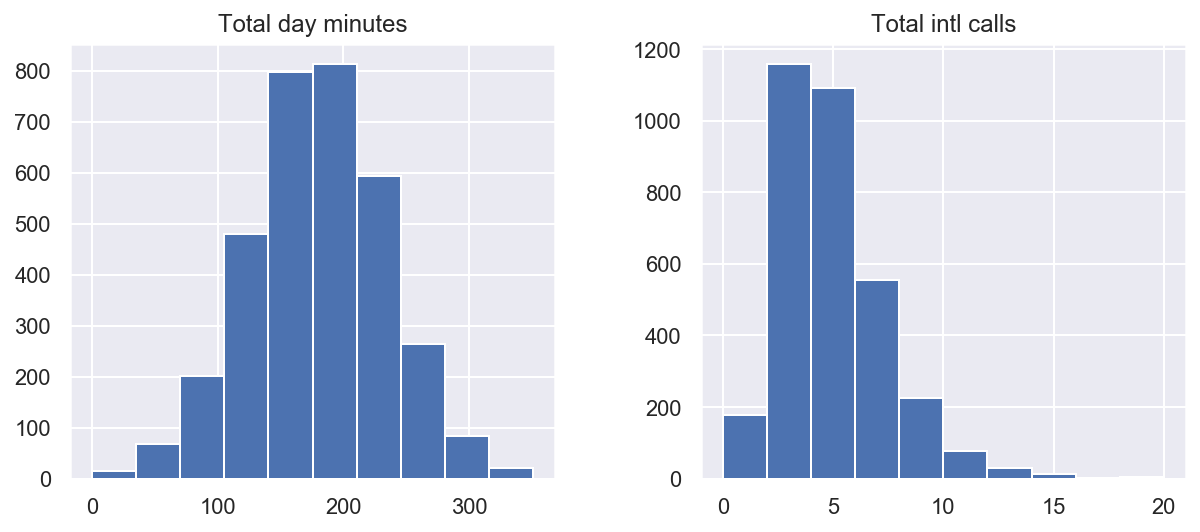A histogram groups values into bins of equal value range. The shape of the histogram may contain clues about the underlying distribution type: Gaussian, exponential, etc. You can also spot any skewness in its shape when the distribution is nearly regular but has some anomalies. Knowing the distribution of the feature values becomes important when you use Machine Learning methods that assume a particular type (most often Gaussian).

In the above plot, we see that the variable Total day minutes is normally distributed, while Total intl calls is prominently skewed right (its tail is longer on the right).

There is also another, often clearer, way to grasp the distribution: density plots or, more formally, Kernel Density Plots. They can be considered a smoothed version of the histogram. Their main advantage over the latter is that they do not depend on the size of the bins. Let's create density plots for the same two variables:

In :
df[features].plot(
kind="density", subplots=True, layout=(1, 2), sharex=False, figsize=(10, 4)
);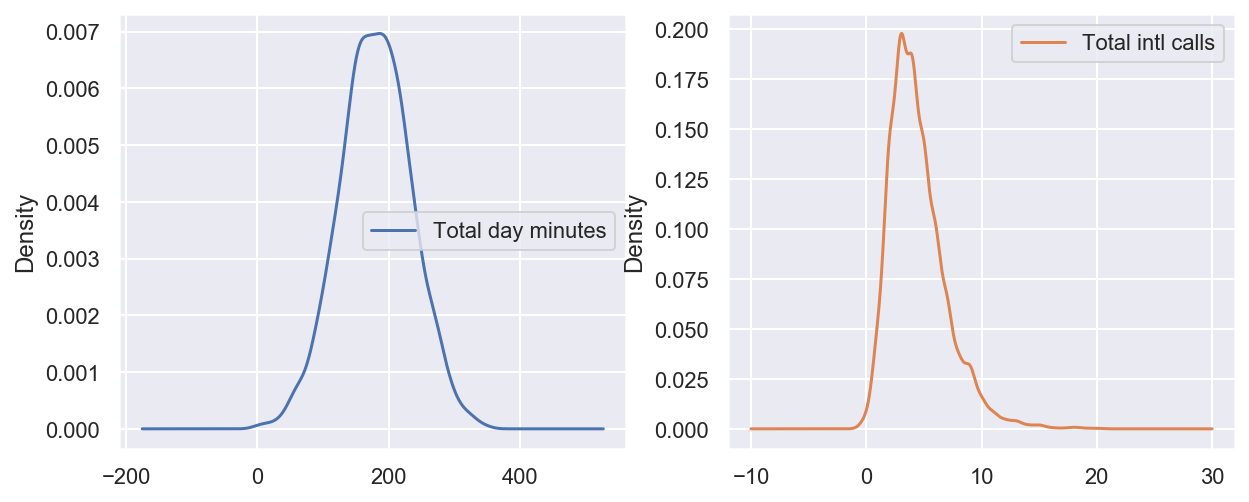It is also possible to plot a distribution of observations with seaborn's distplot(). For example, let's look at the distribution of Total day minutes. By default, the plot displays both the histogram with the kernel density estimate (KDE) on top.

In :
sns.distplot(df["Total intl calls"]);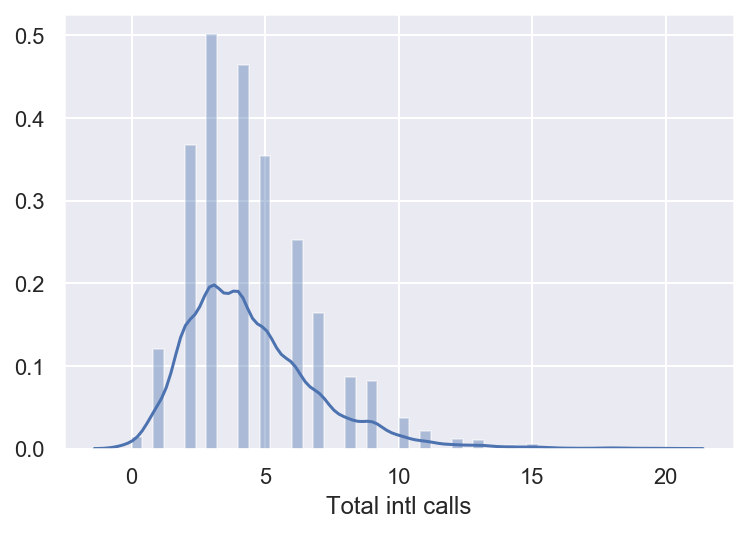The height of the histogram bars here is normed and shows the density rather than the number of examples in each bin.

##### Box plot¶

Another useful type of visualization is a box plot. seaborn does a great job here:

In :
sns.boxplot(x="Total intl calls", data=df);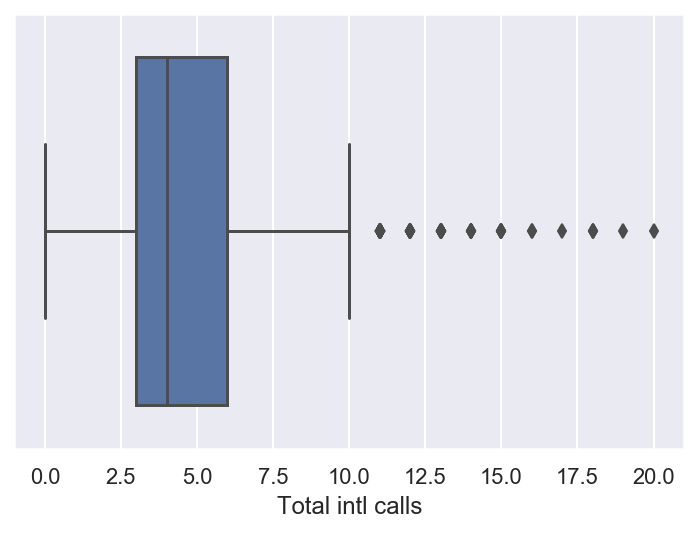Let's see how to interpret a box plot. Its components are a box (obviously, this is why it is called a box plot), the so-called whiskers, and a number of individual points (outliers).

The box by itself illustrates the interquartile spread of the distribution; its length is determined by the $25th \, (\text{Q1})$ and $75th \, (\text{Q3})$ percentiles. The vertical line inside the box marks the median ($50\%$) of the distribution.

The whiskers are the lines extending from the box. They represent the entire scatter of data points, specifically the points that fall within the interval $(\text{Q1} - 1.5 \cdot \text{IQR}, \text{Q3} + 1.5 \cdot \text{IQR})$, where $\text{IQR} = \text{Q3} - \text{Q1}$ is the interquartile range.

Outliers that fall outside of the range bounded by the whiskers are plotted individually as black points along the central axis.

We can see that a large number of international calls is quite rare in our data.

##### Violin plot¶

The last type of distribution plots that we will consider is a violin plot.

Look at the figures below. On the left, we see the already familiar box plot. To the right, there is a violin plot with the kernel density estimate on both sides.

In :
_, axes = plt.subplots(1, 2, sharey=True, figsize=(6, 4))
sns.boxplot(data=df["Total intl calls"], ax=axes)
sns.violinplot(data=df["Total intl calls"], ax=axes);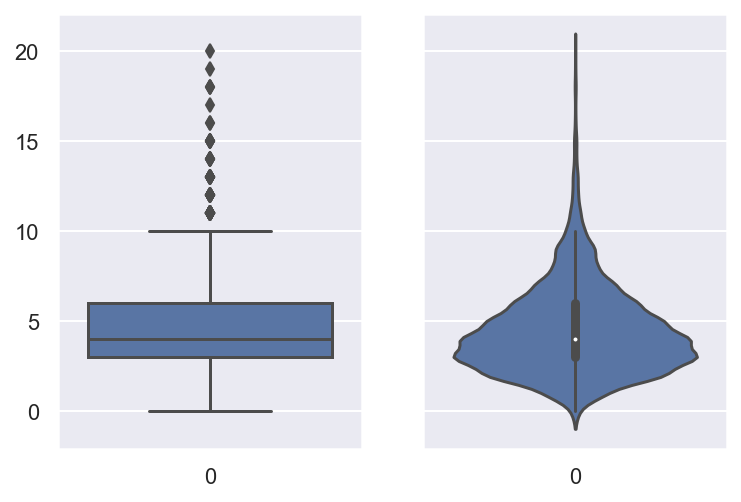The difference between the box and violin plots is that the former illustrates certain statistics concerning individual examples in a dataset while the violin plot concentrates more on the smoothed distribution as a whole.

In our case, the violin plot does not contribute any additional information about the data as everything is clear from the box plot alone.

##### describe()¶

In addition to graphical tools, in order to get the exact numerical statistics of the distribution, we can use the method describe() of a DataFrame:

In :
df[features].describe()

Out:
Total day minutes Total intl calls
count 3333.000000 3333.000000
mean 179.775098 4.479448
std 54.467389 2.461214
min 0.000000 0.000000
25% 143.700000 3.000000
50% 179.400000 4.000000
75% 216.400000 6.000000
max 350.800000 20.000000

Its output is mostly self-explanatory. 25%, 50% and 75% are the corresponding percentiles.

#### 2.2 Categorical and binary features¶

Categorical features take on a fixed number of values. Each of these values assigns an observation to a corresponding group, known as a category, which reflects some qualitative property of this example. Binary variables are an important special case of categorical variables when the number of possible values is exactly 2. If the values of a categorical variable are ordered, it is called ordinal.

##### Frequency table¶

Let's check the class balance in our dataset by looking at the distribution of the target variable: the churn rate. First, we will get a frequency table, which shows how frequent each value of the categorical variable is. For this, we will use the value_counts() method:

In :
df["Churn"].value_counts()

Out:
False    2850
True      483
Name: Churn, dtype: int64

By default, the entries in the output are sorted from the most to the least frequently-occurring values.

In our case, the data is not balanced; that is, our two target classes, loyal and disloyal customers, are not represented equally in the dataset. Only a small part of the clients canceled their subscription to the telecom service. As we will see in the following articles, this fact may imply some restrictions on measuring the classification performance, and, in the future, we may want to additionally penalize our model errors in predicting the minority "Churn" class.

##### Bar plot¶

The bar plot is a graphical representation of the frequency table. The easiest way to create it is to use the seaborn's function countplot(). There is another function in seaborn that is somewhat confusingly called barplot() and is mostly used for representation of some basic statistics of a numerical variable grouped by a categorical feature.

Let's plot the distributions for two categorical variables:

In :
_, axes = plt.subplots(nrows=1, ncols=2, figsize=(12, 4))

sns.countplot(x="Churn", data=df, ax=axes)
sns.countplot(x="Customer service calls", data=df, ax=axes);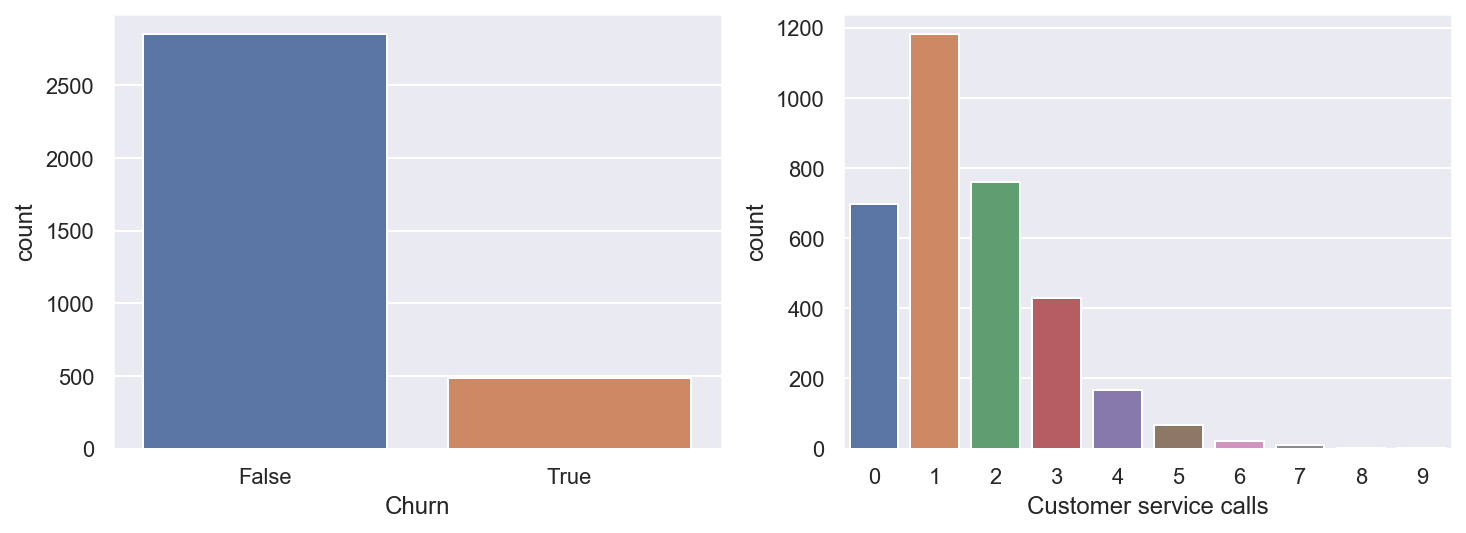While the histograms, discussed above, and bar plots may look similar, there are several differences between them:

1. Histograms are best suited for looking at the distribution of numerical variables while bar plots are used for categorical features.
2. The values on the X-axis in the histogram are numerical; a bar plot can have any type of values on the X-axis: numbers, strings, booleans.
3. The histogram's X-axis is a Cartesian coordinate axis along which values cannot be changed; the ordering of the bars is not predefined. Still, it is useful to note that the bars are often sorted by height, that is, the frequency of the values. Also, when we consider ordinal variables (like Customer service calls in our data), the bars are usually ordered by variable value.

The left chart above vividly illustrates the imbalance in our target variable. The bar plot for Customer service calls on the right gives a hint that the majority of customers resolve their problems in maximum 2-3 calls. But, as we want to be able to predict the minority class, we may be more interested in how the fewer dissatisfied customers behave. It may well be that the tail of that bar plot contains most of our churn. These are just hypotheses for now, so let's move on to some more interesting and powerful visual techniques.

## 3. Multivariate visualization¶

Multivariate plots allow us to see relationships between two and more different variables, all in one figure. Just as in the case of univariate plots, the specific type of visualization will depend on the types of the variables being analyzed.

#### 3.1 Quantitative vs. Quantitative¶

##### Correlation matrix¶

Let's look at the correlations among the numerical variables in our dataset. This information is important to know as there are Machine Learning algorithms (for example, linear and logistic regression) that do not handle highly correlated input variables well.

First, we will use the method corr() on a DataFrame that calculates the correlation between each pair of features. Then, we pass the resulting correlation matrix to heatmap() from seaborn, which renders a color-coded matrix for the provided values:

In :
# Drop non-numerical variables
numerical = list(
set(df.columns)
- {
"State",
"International plan",
"Voice mail plan",
"Area code",
"Churn",
"Customer service calls",
}
)

# Calculate and plot
corr_matrix = df[numerical].corr()
sns.heatmap(corr_matrix);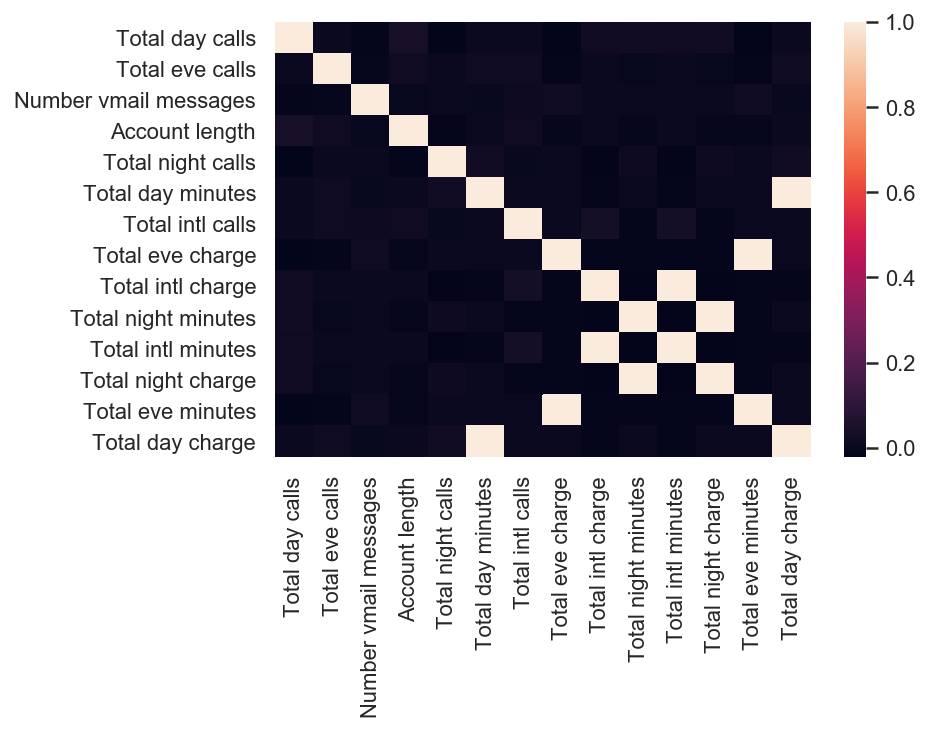From the colored correlation matrix generated above, we can see that there are 4 variables such as Total day charge that have been calculated directly from the number of minutes spent on phone calls (Total day minutes). These are called dependent variables and can therefore be left out since they do not contribute any additional information. Let's get rid of them:

In :
numerical = list(
set(numerical)
- {
"Total day charge",
"Total eve charge",
"Total night charge",
"Total intl charge",
}
)

##### Scatter plot¶

The scatter plot displays values of two numerical variables as Cartesian coordinates in 2D space. Scatter plots in 3D are also possible.

Let's try out the function scatter() from the matplotlib library:

In :
plt.scatter(df["Total day minutes"], df["Total night minutes"]);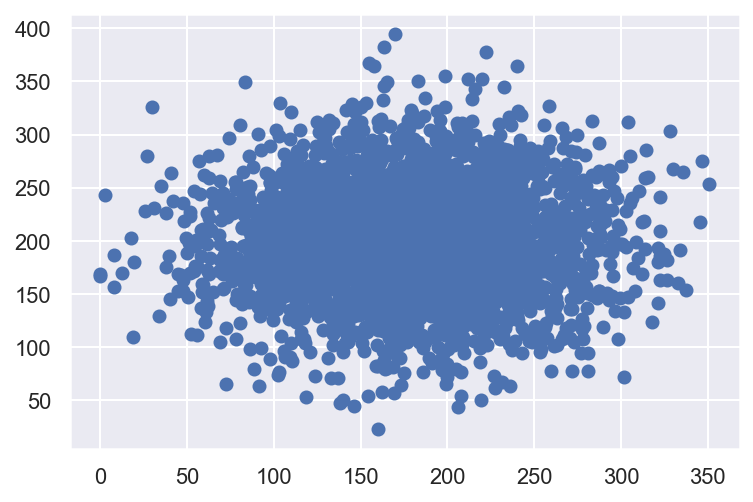We get an uninteresting picture of two normally distributed variables. Also, it seems that these features are uncorrelated because the ellipse-like shape is aligned with the axes.

There is a slightly fancier option to create a scatter plot with the seaborn library:

In :
sns.jointplot(x="Total day minutes", y="Total night minutes", data=df, kind="scatter");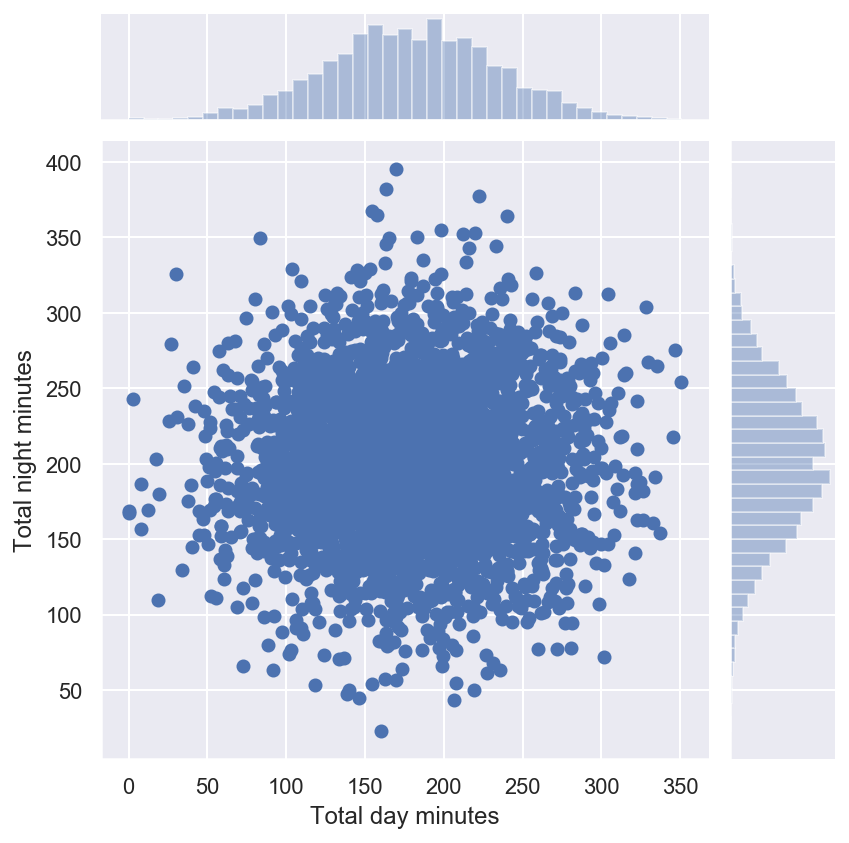The function jointplot() plots two histograms that may be useful in some cases.

Using the same function, we can also get a smoothed version of our bivariate distribution:

In :
sns.jointplot(
"Total day minutes", "Total night minutes", data=df, kind="kde", color="g"
);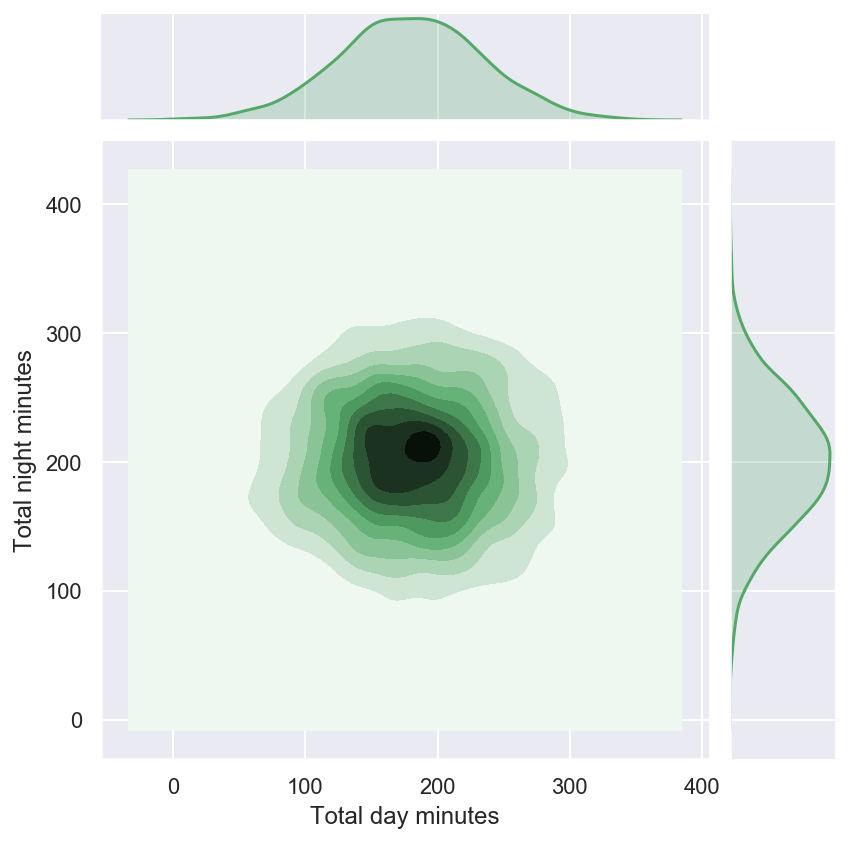This is basically a bivariate version of the Kernel Density Plot discussed earlier.

##### Scatterplot matrix¶

In some cases, we may want to plot a scatterplot matrix such as the one shown below. Its diagonal contains the distributions of the corresponding variables, and the scatter plots for each pair of variables fill the rest of the matrix.

In :
# pairplot() may become very slow with the SVG or retina format
%config InlineBackend.figure_format = 'png'
sns.pairplot(df[numerical]);